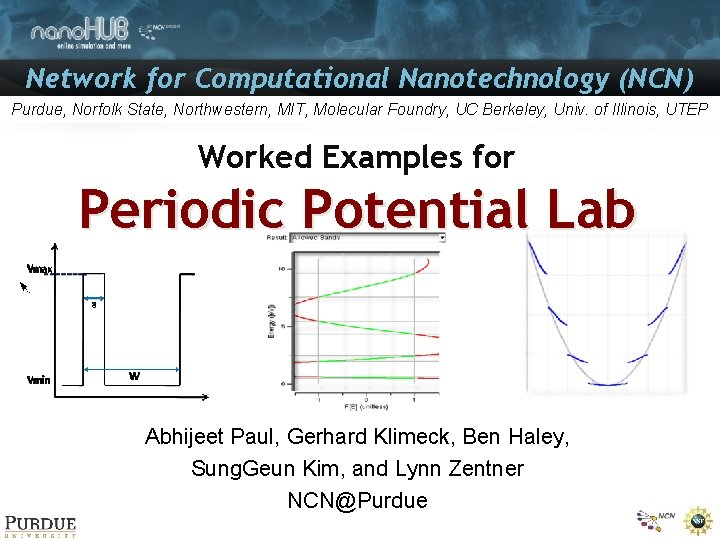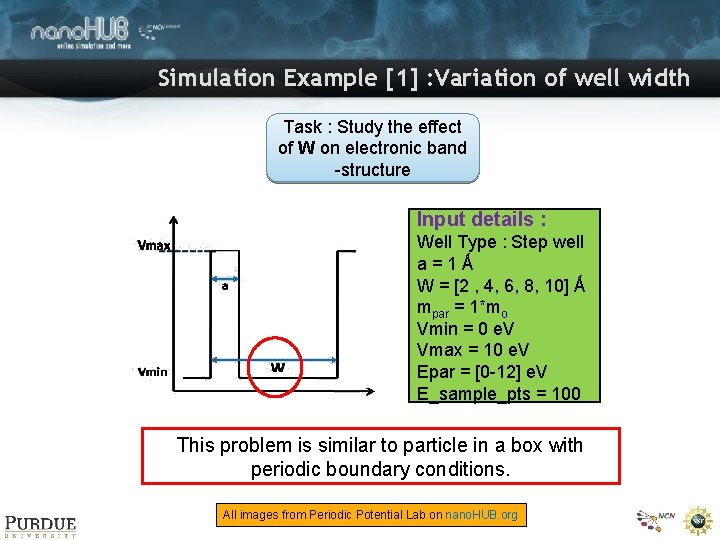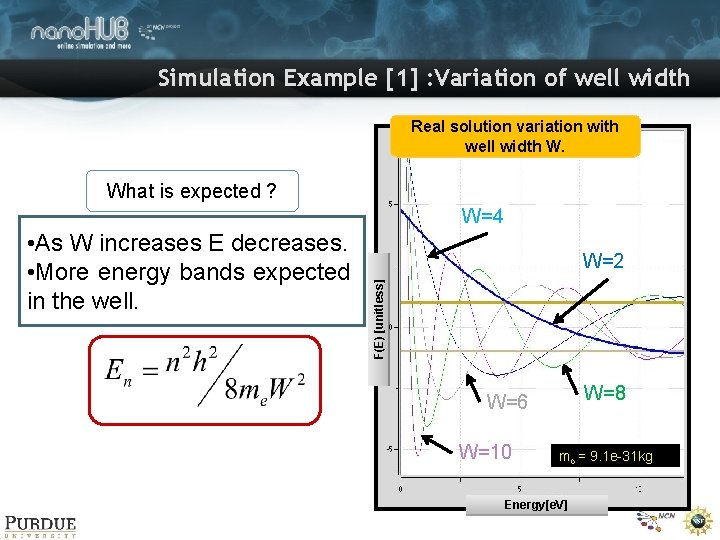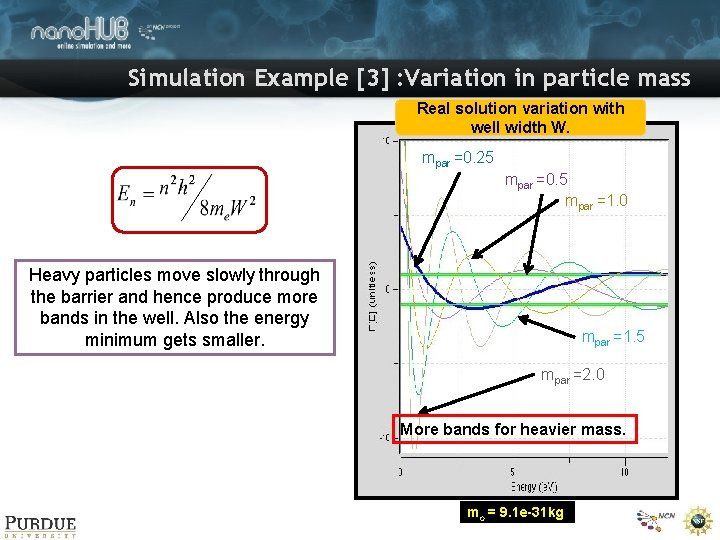# Network for Computational Nanotechnology NCN Purdue Norfolk State

• Slides: 7Network for Computational Nanotechnology (NCN) Purdue, Norfolk State, Northwestern, MIT, Molecular Foundry, UC Berkeley, Univ. of Illinois, UTEP Worked Examples for Periodic Potential Lab Abhijeet Paul, Gerhard Klimeck, Ben Haley, Sung. Geun Kim, and Lynn Zentner [email protected]Simulation Example  : Variation of well width Task : Study the effect of W on electronic band -structure Input details : Well Type : Step well a=1Ǻ W = [2 , 4, 6, 8, 10] Ǻ mpar = 1*mo Vmin = 0 e. V Vmax = 10 e. V Epar = [0 -12] e. V E_sample_pts = 100 This problem is similar to particle in a box with periodic boundary conditions. All images from Periodic Potential Lab on nano. HUB. orgSimulation Example  : Variation of well width Real solution variation with well width W. What is expected ? W=4 W=2 F(E) [unitless] • As W increases E decreases. • More energy bands expected in the well. W=8 W=6 W=10 mo = 9. 1 e-31 kg Energy[e. V]Simulation Example  : Variation of well width Lowest energy band for different well widths. E(min) ~ 1/W 2 Bandwidth W=2 W=10 W=4 W=6 W=8 Well No. of Minimum Width bands energy (A) (e. V) Band width (e. V) Band gap (e. V) 2 1 4. 51 6. 358 -- 4 2 1. 61 0. 908 3. 725 6 3 0. 814 0. 275 2. 119 8 4 0. 489 0. 116 1. 336 10 5 0. 326 0. 058 0. 912 Table showing some of the important band parameters All images from Periodic Potential Lab on nano. HUB. orgSimulation Example  : Variation in particle mass Task : Study the effect of mpar on electronic band-structure Input details : Well Type : Step well a=1Ǻ W=8Ǻ mpar = [0. 25, 0. 5, 1, 1. 5, 2]*mo Vmin = 0 e. V Vmax = 10 e. V Epar = [0 -12] e. V E_sample_pts = 100 All images from Periodic Potential Lab on nano. HUB. orgSimulation Example  : Variation in particle mass Real solution variation with well width W. mpar =0. 25 mpar =0. 5 mpar =1. 0 Heavy particles move slowly through the barrier and hence produce more bands in the well. Also the energy minimum gets smaller. mpar =1. 5 mpar =2. 0 More bands for heavier mass. mo = 9. 1 e-31 kgSimulation Example  : Variation in particle mass m=2. 0 m=1. 5 m=1. 0 m=0. 50 m=0. 25 Transmission of a particle through the well. A heavier particle has limited transmission. A lighter particle easily passes through the barrier. m (mo) No. of bands Minimum energy (e. V) Band width (e. V) Band gap (e. V) 0. 25 2 0. 961 1. 362 2. 175 0. 50 3 0. 70 0. 51 1. 77 1. 0 4 0. 489 0. 116 1. 336 1. 5 5 0. 365 0. 046 1. 036 2. 0 6 0. 29 0. 023 0. 842 Table showing some of the important band parameters All images from Periodic Potential Lab on nano. HUB. org